The NIST reference dataset contains 27 datasets for validating nonlinear regression analysis software. The datasets were distributed by level of difficulty: 8 lower, 11 average and 8 higher. Here, will be presented the LAB Fit results for the higher level. For each dataset a function is indicated and the initial values are suggested (two groups). The two groups were tested by LAB Fit. For most of the datasets, the uncertainty could be given with 1 or 2 significant digits. However, the LAB Fit results were presented with 3 digits for the uncertainty. The value 1 was assumed for the "POWER" parameter. If there was not success, another value was specified. The default value for the tolerance (1.0E-06) was assumed. Although LAB Fit calculates the covariance matrix (for error propagation purposes, confidence and prediction bands), the results won't be presented because the SRD / NIST doesn't certify it.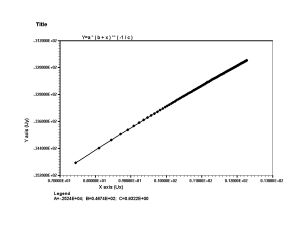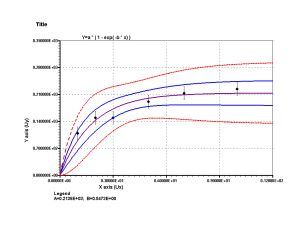Confidence and prediction bands, error bars and extrapolation.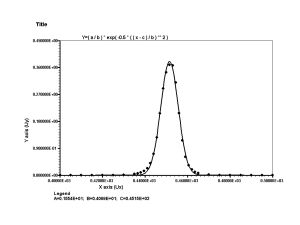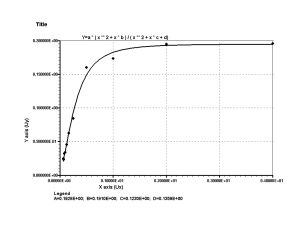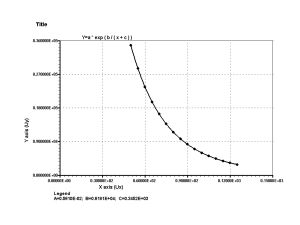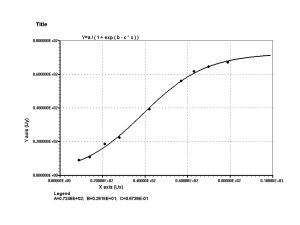Extrapolation.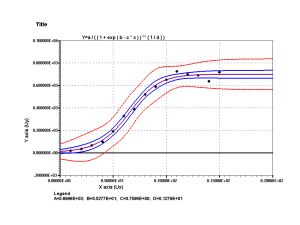Confidence and prediction bands and extrapolation.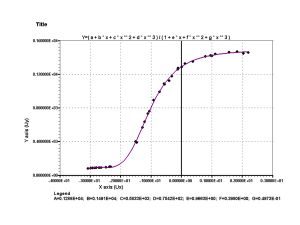BENNETT5 - Dataset, initial and certified values                             y = a * ( b + x ) ** ( -1 / c ) a = -2524 Ý 297 b = 46.74 Ý 1.25 c = 0.9322 Ý 0.0203 Obs: the results agree with the validated values by the SRD of the NIST (initial values suggested: primary [POWER = 300] and secondary [POWER = 300]). BOXBOD - Dataset, initial and certified values y = a * ( 1 - exp( -b * x) ) a = 213.8 Ý 12.4 b = 0.547 Ý 0.105 Observation:     the results agree with the validated values by the SRD of the NIST (initial values suggested: primary and secondary groups). ECKERLE4 - Dataset, initial and certified values y = ( a / b ) * exp( -0.5 * ( ( x - c ) / b ) ** 2 ) a = 1.5544 Ý 0.0154 b = 4.0888 Ý 0.0468 c = 451.5412 Ý 0.0468 Observation:     the results agree with the validated values by the SRD of the NIST (initial values suggested: primary and secondary groups). MGH09 - Dataset, initial and certified values y = a * ( x ** 2 + x * b ) / ( x ** 2 + x * c + d) a = 0.1928 Ý 0.0114 b = 0.191 Ý 0.196 c = 0.1231 Ý 0.0809 d = 0.1361 Ý 0.0900 Observation:     the results agree with the validated values by the SRD of the NIST (initial values suggested: primary [POWER = 10000] and secondary). MGH10 - Dataset, initial and certified values y = a * exp ( b / ( x + c ) ) a = 0.005610 Ý 0.000157 b = 6181.3 Ý 23.3 c = 345.224 Ý 0.785 Observation:     the results agree with the validated values by the SRD of the NIST (initial values suggested: primary [POWER = 10000] and secondary). RAT42 - Dataset, initial and certified values y = a / ( 1 + exp ( b - c * x ) ) a = 72.46 Ý 1.73 b = 2.6181 Ý 0.0883 c = 0.06736 Ý 0.00345 Observation:     the results agree with the validated values by the SRD of the NIST (initial values suggested: primary and secondary groups). RAT43 - Dataset, initial and certified values y = a / ( ( 1 + exp ( b - c * x ) ) ** ( 1 / d ) ) a = 699.6 Ý 16.3 b = 5.28 Ý 2.08 c = 0.760 Ý 0.196 d = 1.279 Ý 0.688 Observation:     the results agree with the validated values by the SRD of the NIST (initial values suggested: primary and secondary groups). THURBER - Dataset, initial and certified values y = ( a + b * x + c * x ** 2 + d * x ** 3 ) / ( 1 + e * x + f * x ** 2 + g * x ** 3 ) a = 1288.14 Ý 4.66   b = 1491.1 Ý 39.6 c = 583.3 Ý 28.7   d = 75.42 Ý 5.57 e = 0.9663 Ý 0.0313   f = 0.3980 Ý 0.0150 g = 0.04973 Ý 0.00658 Observation:     the results agree with the validated values by the SRD of the NIST (initial values suggested: primary and secondary groups;   tolerance = 1.0E-08). Top All the LAB Fit results are statistically correct...                                   For all the initial values...

| Back |